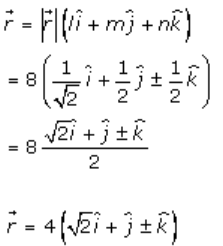# RD Sharma Solutions Class 12 Algebra Of Vectors Exercise 23.9

RD Sharma Solutions for Class 12 Maths Chapter 23 Algebra of Vectors Exercise 23.9 are given here to improve the problem-solving skills of the students. These solutions are developed by subject experts according to the latest CBSE guidelines so that students can achieve high marks in their exams. The RD Sharma Class 12 Solutions is the right resource to kick start preparations for the board exams. Students can now download the PDF containing the solutions for this exercise effortlessly, from the link provided below.

## Download PDF of Rd Sharma Solution for Class 12 Maths Chapter 23 Exercise 9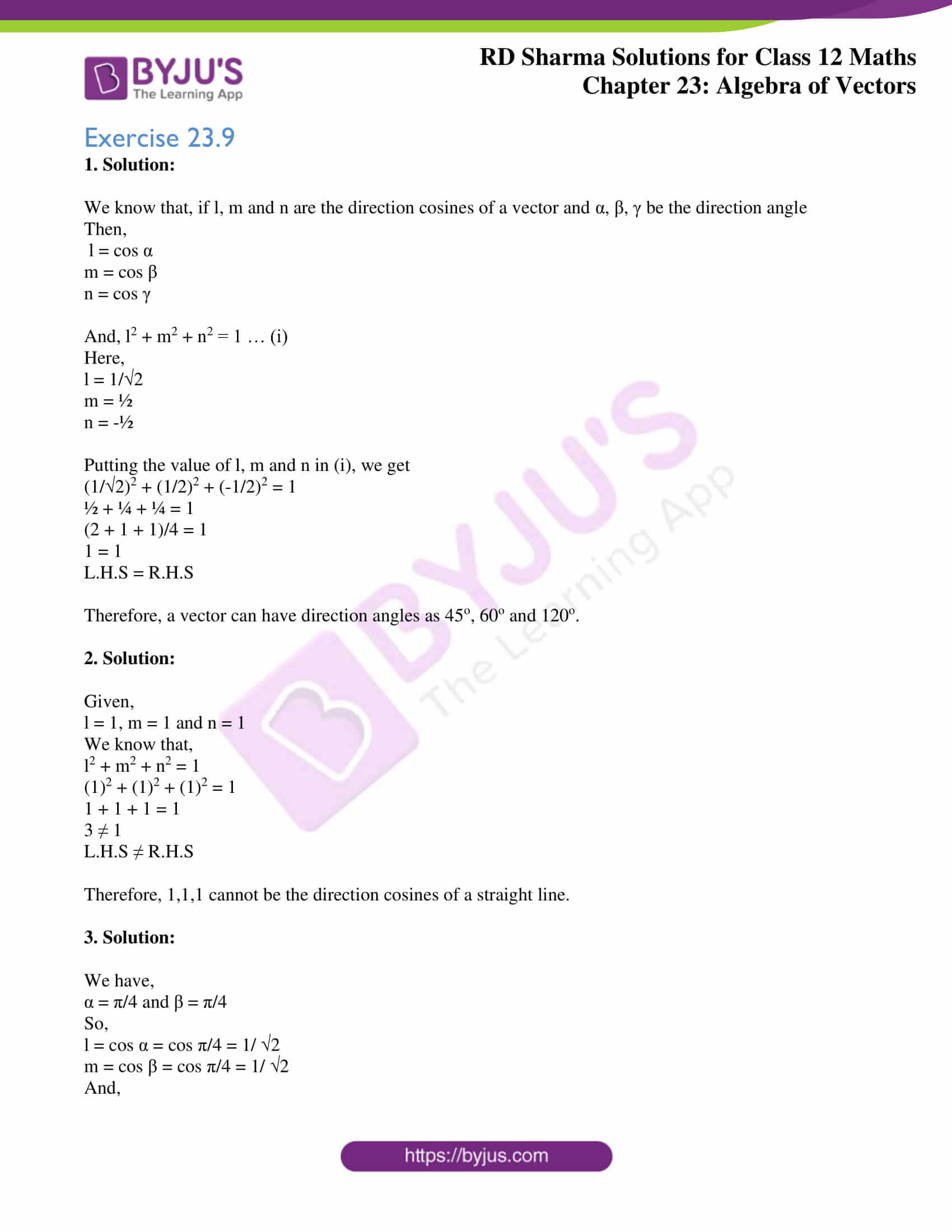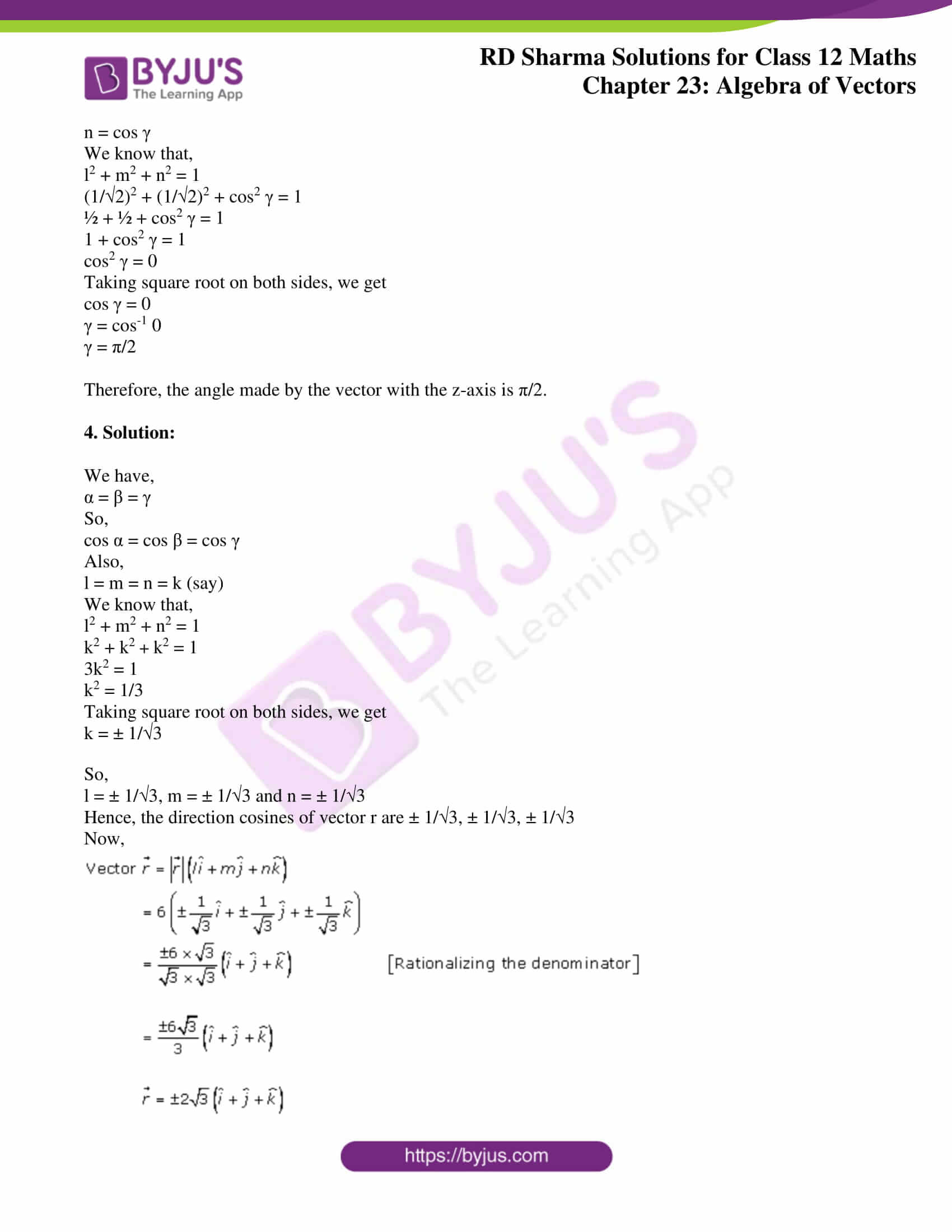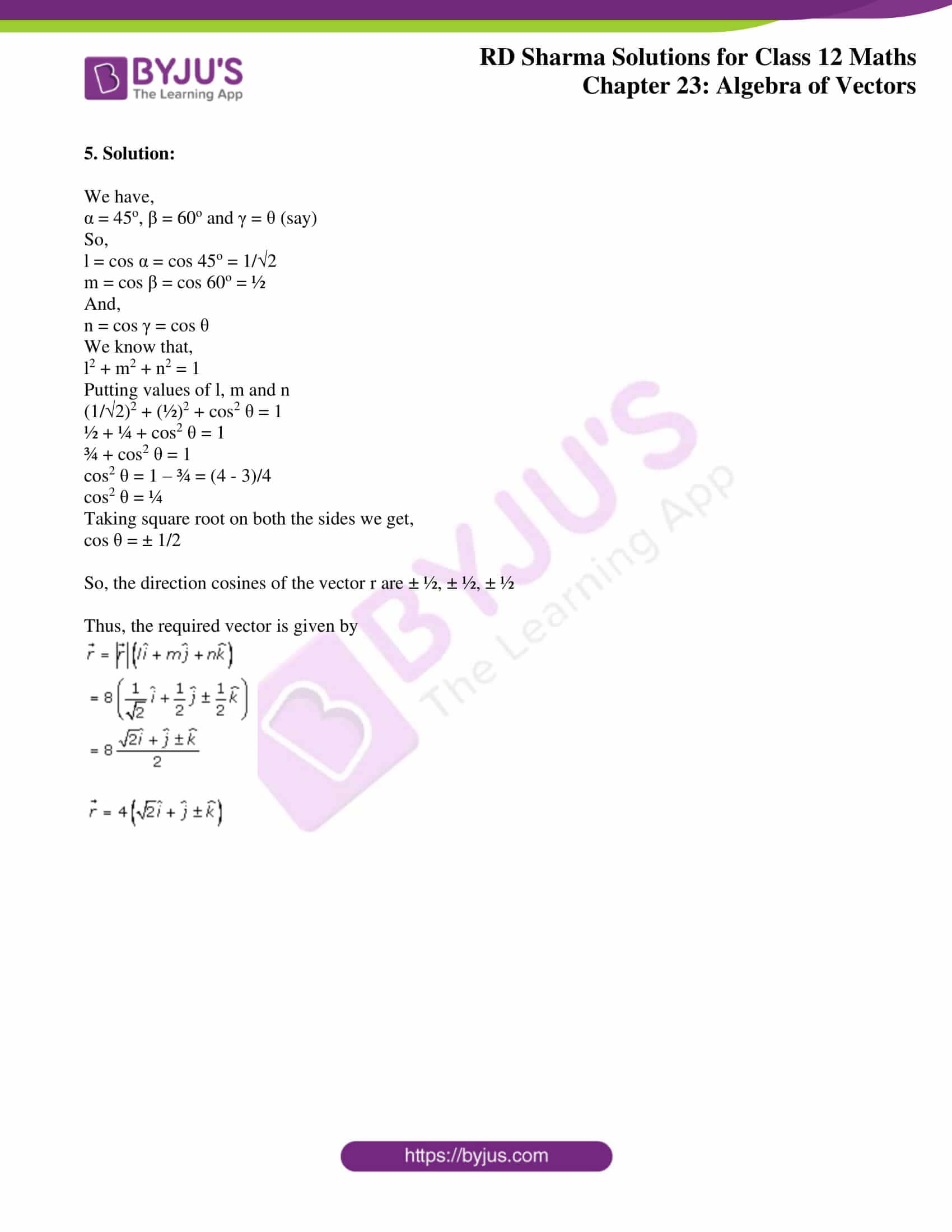### Access Answers for Rd Sharma Solution Class 12 Maths Chapter 23 Exercise 9

Exercise 23.9

1. Solution:

We know that, if l, m and n are the direction cosines of a vector and α, β, γ be the direction angle

Then,

l = cos α

m = cos β

n = cos γ

And, l2 + m2 + n2 = 1 … (i)

Here,

l = 1/√2

m = ½

n = -½

Putting the value of l, m and n in (i), we get

(1/√2)2 + (1/2)2 + (-1/2)2 = 1

½ + ¼ + ¼ = 1

(2 + 1 + 1)/4 = 1

1 = 1

L.H.S = R.H.S

Therefore, a vector can have direction angles as 45o, 60o and 120o.

2. Solution:

Given,

l = 1, m = 1 and n = 1

We know that,

l2 + m2 + n2 = 1

(1)2 + (1)2 + (1)2 = 1

1 + 1 + 1 = 1

3 ≠ 1

L.H.S ≠ R.H.S

Therefore, 1,1,1 cannot be the direction cosines of a straight line.

3. Solution:

We have,

α = π/4 and β = π/4

So,

l = cos α = cos π/4 = 1/ √2

m = cos β = cos π/4 = 1/ √2

And,

n = cos γ

We know that,

l2 + m2 + n2 = 1

(1/√2)2 + (1/√2)2 + cos2 γ = 1

½ + ½ + cos2 γ = 1

1 + cos2 γ = 1

cos2 γ = 0

Taking square root on both sides, we get

cos γ = 0

γ = cos-1 0

γ = π/2

Therefore, the angle made by the vector with the z-axis is π/2.

4. Solution:

We have,

α = β = γ

So,

cos α = cos β = cos γ

Also,

l = m = n = k (say)

We know that,

l2 + m2 + n2 = 1

k2 + k2 + k2 = 1

3k2 = 1

k2 = 1/3

Taking square root on both sides, we get

k = ± 1/√3

So,

l = ± 1/√3, m = ± 1/√3 and n = ± 1/√3

Hence, the direction cosines of vector r are ± 1/√3, ± 1/√3, ± 1/√3

Now,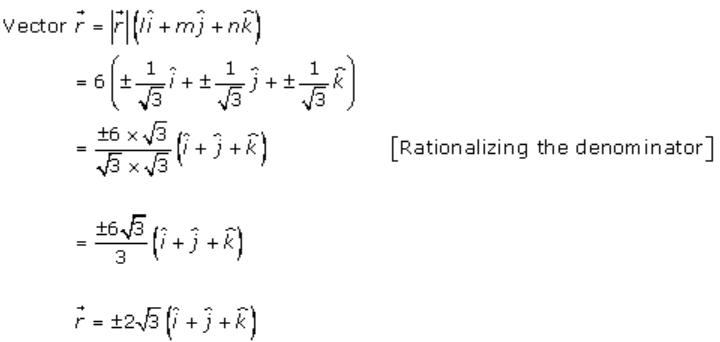5. Solution:

We have,

α = 45o, β = 60o and γ = θ (say)

So,

l = cos α = cos 45o = 1/√2

m = cos β = cos 60o = ½

And,

n = cos γ = cos θ

We know that,

l2 + m2 + n2 = 1

Putting values of l, m and n

(1/√2)2 + (½)2 + cos2 θ = 1

½ + ¼ + cos2 θ = 1

¾ + cos2 θ = 1

cos2 θ = 1 – ¾ = (4 – 3)/4

cos2 θ = ¼

Taking square root on both the sides we get,

cos θ = ± 1/2

So, the direction cosines of the vector r are ± ½, ± ½, ± ½

Thus, the required vector is given by